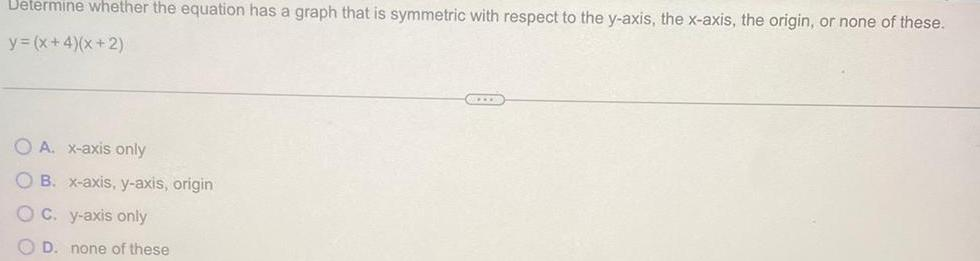Question:

# Determine whether the equation has a graph that is symmetric

Last updated: 11/11/2023Determine whether the equation has a graph that is symmetric with respect to the y axis the x axis the origin or none of these y x 4 x 2 OA x axis only OB x axis y axis origin OC y axis only OD none of these ALLEN